Select and Omit criteria in Logical file in AS400 - AS400 and SQL Tricks

# Select and Omit criteria in Logical file in AS400

Select and Omit criteria in Logical file in AS400Select and Omit criteria in Logical file in AS400

## Introduction

Select and Omit criteria are specified on Logical files over the physical files to filter out the data from the PF as per defined criteria.

First, Let's create a physical file (PF) named PF1

``````
A          R FMT1
A            NAME          20A
A            AGE            3P 0
A            COUNT          3P 0
``````

Create the PF named PF1 using command CRTPF and then insert records as below.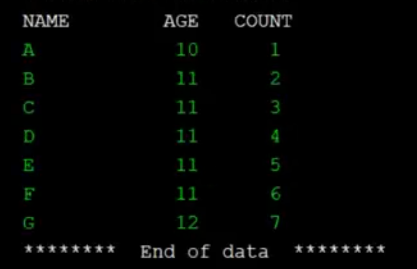PF1 records

## Example 1

Let's create Logical file LF1 on the Physical file PF1.

``````
A          R FMT1                      PFILE(PF1)
A          K COUNT
A          S COUNT                     CMP(EQ 5)
``````

In LF1, we only select a record that is compared equals 5. Let's create the Logical file (LF) using the command CRTLF. Please ensure that the library having PF1 must be in the library list before compiling LF and creating its object.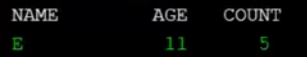LF1 records

## Example 2

Let's create Logical file LF2 on the Physical file PF1.

``````
A          R FMT1                      PFILE(PF1)
A          K COUNT
A          S COUNT                     CMP(GE 5)
``````

In LF2, we only select the records that are greater equals 5.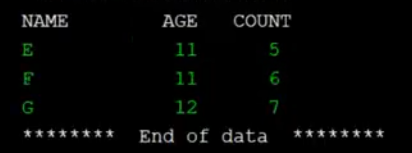LF2 records

## Example 3

Let's create Logical file LF3 on the Physical file PF1.

``````
A          R FMT1                      PFILE(PF1)
A          K COUNT
A          S COUNT                     CMP(GT 5)
``````

In LF3, we only select the records that are greater than 5.LF3 records

## Example 4

Let's create Logical file LF4 on the Physical file PF1.

``````
A          R FMT1                      PFILE(PF1)
A          K COUNT
A          S COUNT                     CMP(LE 5)
``````

In LF4, we only select the records that are less than equals to 5.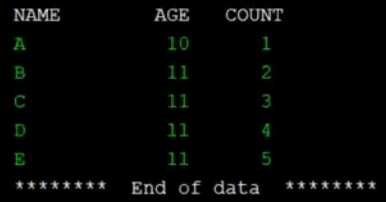LF4 records

## Example 5

Let's create Logical file LF5 on the Physical file PF1.

``````
A          R FMT1                      PFILE(PF1)
A          K COUNT
A          S COUNT                     CMP(LT 5)
``````

In LF5, we only select the records that are less than 5.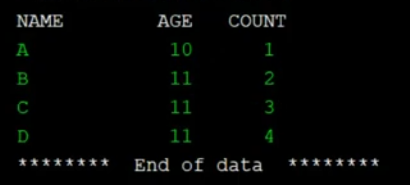LF5 records

## Example 6

Let's create Logical file LF6 on the Physical file PF1.

``````
A          R FMT1                      PFILE(PF1)
A          K COUNT
A          S COUNT                     CMP(NG 5)
``````

In LF6, we only select the records that are not greater than 5.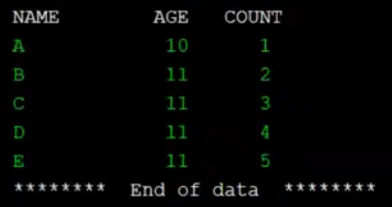LF6 records

## Example 7

Let's create Logical file LF7 on the Physical file PF1.

``````
A          R FMT1                      PFILE(PF1)
A          K COUNT
A          S COUNT                     CMP(NL 5)
``````

In LF7, we only select the records that are not less than 5.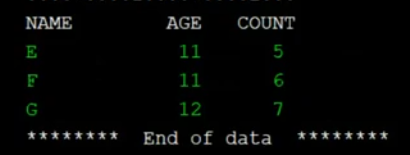LF7 records

## Example 8

Let's create Logical file LF8 on the Physical file PF1.

``````
A          R FMT1                      PFILE(PF1)
A          K COUNT
A          S COUNT                     CMP(NE 5)
``````

In LF8, we only select the records that are not equal to 5.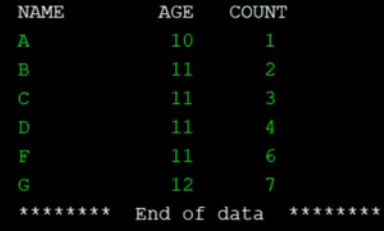LF8 records

## Example 9

Let's create Logical file LF9 on the Physical file PF1.

``````
A          R FMT1                      PFILE(PF1)
A          K COUNT
A          S COUNT                     RANGE(3 5)
``````

In LF9, we only select the records that are only within the range of 3 to 5.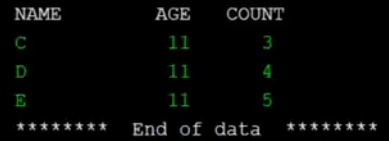LF9 records

## Example 10

Let's create Logical file LF10 on the Physical file PF1.

``````
A          R FMT1                      PFILE(PF1)
A          K COUNT
A          S COUNT                     VALUES(1 2 3)
``````

In LF10, we only select the records that have values 1 or 2 or 3.LF10 records

## Example 11

Let's create Logical file LF11 on the Physical file PF1.

``````
A          R FMT1                      PFILE(PF1)
A          K NAME
A          K COUNT
A          S NAME                      VALUES('A' 'B')
``````

In LF11, we only select the records that have values A or B for the Name field.LF11 records

## Example 12

Let's create Logical file LF12 on the Physical file PF1.

``````
A          R FMT1                      PFILE(PF1)
A          K NAME
A          K COUNT
A          S NAME                      VALUES('A' 'B')
A            COUNT                     COMP(GT 5)
``````

In LF12, we select the records that have values A or B for the Name field and the count is greater than 5 for the COUNT field.LF12 records

## Example 13

Let's create Logical file LF13 on the Physical file PF1.

``````
A          R FMT1                      PFILE(PF1)
A          K NAME
A          K COUNT
A          S NAME                      VALUES('A' 'B')
A          O COUNT                     COMP(GT 5)
``````

In LF13 we select the records that have values A or B for the Name field and omit the record having a count is greater than 5 for the COUNT field.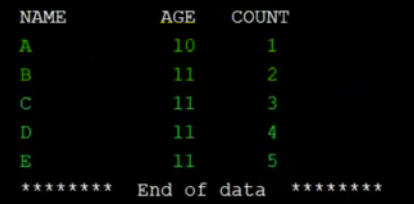LF13 records

## Example 14

Let's create Logical file LF14 on the Physical file PF1.

``````
A          R FMT1                      PFILE(PF1)
A          K NAME
A          K COUNT
A          S NAME                      VALUES('A' 'B')
A          O                           ALL
``````

In LF14 we select the records that have values A or B for the Name field and omit All other records.LF14 records

## Example 15

Let's create Logical file LF15 on the Physical file PF1.

``````
A          R FMT1                      PFILE(PF1)
A          K NAME
A          K AGE
A          S NAME                      VALUES('A' 'B')
A            AGE                       COMP(EQ 10)
``````

In LF15 we select the records that have values A or B for the Name field and also have AGE equals 10.LF15 records